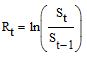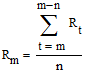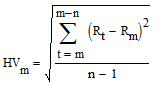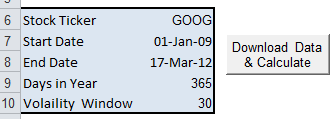# Calculate Historical Volatility in Excel

This spreadsheet calculates the historical volatility of a stock. It uses returns data automatically downloaded from Yahoo.

Historical volatility is the standard deviation of an asset’s historical returns. The standard deviation is calculated over a moving time window.

The historical volatility of a stock is distinct from implied volatility of an option. The former represents past movements in price. The latter represents future expectations about price movements, and is calculated from the option price.

By comparing the historical volatility of the underlying to the implied volatility of the option, investors can judge if the option is cheap or expensive. If the implied volatility is high, then selling the option is sensible.  However, if the implied volatility is low, the option is a good buy.

## How to Calculate Historical Volatility

1. Calculate the natural log of the current stock price to yesterday’s stock price. This is the continuously compounded return.2. Calculate the average return over a moving time window of n days. A value of n = 21 represent the typical number of trading days in a month, and is often used. Values lower than this tend to produce lots of noise in the results. The greater the time window, the smoother the results.3. Calculate the standard deviation of the returns over the moving time window.4. Annualize the daily standard deviation by multiplying by the square root of the number of days in a year. The average number of trading days in a year is 252.

## Calculate Historical Volatility in Excel

The spreadsheet automates the steps described above, and is simple to use. Simply enter the stock ticker, the start and end dates, and the volatility window (i.e. the number of days over which the volatility is calculated).The end date is set to NOW() by default, which gives the current date.

After clicking the button, the spreadsheet downloads returns data from Yahoo using VBA. Then, the chart will plot the historical volatility (based on the daily adjusted close)### 21 thoughts on “Calculate Historical Volatility in Excel”

1. Hi, This has been wroking fine until after the 4th July, recently, I could no longer download the data for Dow Jones(^DJI) but other still work fine with other data, e,g, FTSE, DAX, which I use daily. Could someone into look at this? Many thanks. T.

2. Please ignore request as I’ve fixed the problem… yahoo went and changed the stock ticker – it’s now DJIA.

3. Great tool but unfortunately in Excel for Mac 2011 there is a VBS error message. Somehow your other excel files that run on Excel 2002 work.

Is there a work around or another version of this worksheet that would work in Mac?

Thanks!

4. I have a question, how do I read this chart? What does it mean if the voltaitly is high and the adjusted close is low? Am I looking for when they are close to one another?

great app by the way!

5. This Excel file will not run on MS Office 2010.

• I’ve just run this Excel spreadsheet on Excel 2010 and Windows 7 64bit. Works for me.

6. Hello Samir,

You have calculated Standard Deviation (Volatility) & Histogram for a single Stock. How it can be calculated for multiple number of stocks?

7. HELLO,SAMIR

CN U PREPARE SAME HISTORICAL VOLATILITY CHART FOR “NSE SCRIPTS N INDEXES OF INDIA”…..

IT WILL B GREAT HELP 4 ME….

I SEARCH EVERY CORNER OF INTERNET,BT INDIAN OPTIONS HV IS NOT AVAILABLE

PLS HELP

REGARDS

• Hello Ajay ,

This Excel Sheet created by Samir works perfectly with NSE Stocks. Only thing is that you have to change the Ticker name to NSE Stock Ticker. In Yahoo if you want ticker symbol of State bank of India then you have to write like this SBIN.NS . Thats it . For the whole list of symbols you may refer to the undermentioned link:

Yahoo NSE Symbol List

8. In the spreadsheet, how come the closing prices are used to compute standard deviation, instead of using ADJUSTED closing prices. Wouldn’t the adjusted closing price be more accurate, since it takes into account share splits and dividends?

• It’s simply a matter of choice and preference. No other reason.

9. This is a good introduction. I have a question about using closing price or adjusted price. If we want to calculate volatility for OPTION PRICING, which one is more appropriate? It seems that closing price is more suitable in this situation? What is your opinion? Any reference?

10. Thanks for the simple explanation.
Based on this logic, how can i forecast the distribution of the price of a commodity n days from now, if i know the price now. Say with 1 sigma Conf Interval.

11. Hello Everyone,

I want to calculate the Series of standard deviation of annual growth rate of GDP (1970-2013). Can anyone help please??

12. Will this work for NSE stocks in India.Doesn’t seem so as of now.

13. Hi Samir,
Seems the macro can’t be viewed because there is a password.

14. Dear Samir,

A simple question about the “days in Year calculation”.
From where we got 252 days, I tried to substract the starting date from the ending date, but the result was 242.

15. Do you know if this software is compatible with Open Office — which is often compatible with Excel

• I don’t know. Never tried. If you do, let me know.

Thanks